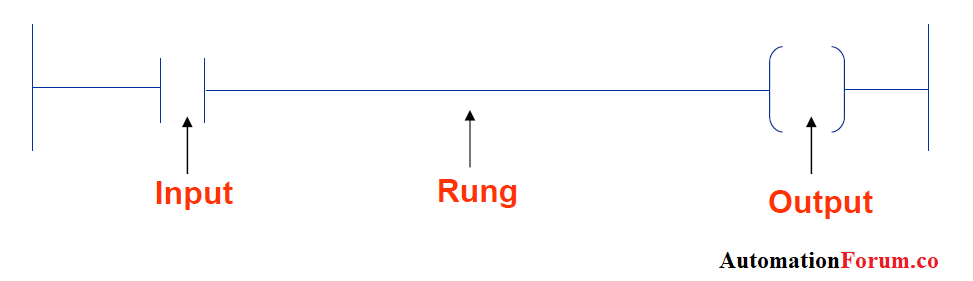# How to write a PLC ladder logic program?The ladder logic programming is commonly used to program PLCs. A PLC takes input from field devices and based on the programming instructions controls output devices. Writing a program is then equivalent to drawing a switching circuit. The ladder diagram consists of two vertical lines representing the power rails. Circuits are connected as horizontal lines, i.e., the rungs of the ladder, between these two verticals.

Below figure illustrates a simple ladder diagram:• The vertical lines of the diagram represent the power rails between which circuits are connected. The power flow is taken to be from the left-hand vertical across a rung. Each rung on the ladder defines one operation in the control process.
• Each Rung of a ladder logic diagram represents a condition of the input/output/ memory bits. Each rung can contain more than one input in different configurations as shown below:• A ladder diagram is read from left to right and from top to bottom,  The top rung is read from
left to right. Then the second rung down is read from left to right and so on, as the figure shows:• Each row must begin with an entry or entries and must end with at least one exit. The term input is used for a control action, such as closing the contacts of a switch, used as an input to the PLC. The term output is used for a device connected to the output of a PLC, for example, a motor.
• A particular device can appear in more than one rung of a ladder.
• The inputs and outputs are all identified by their addresses, the notation used depending on the PLC manufacturer.

The figure below shows the input and output configurations in ladder logic programs:A simple conversion for relay logic to ladder logic example: